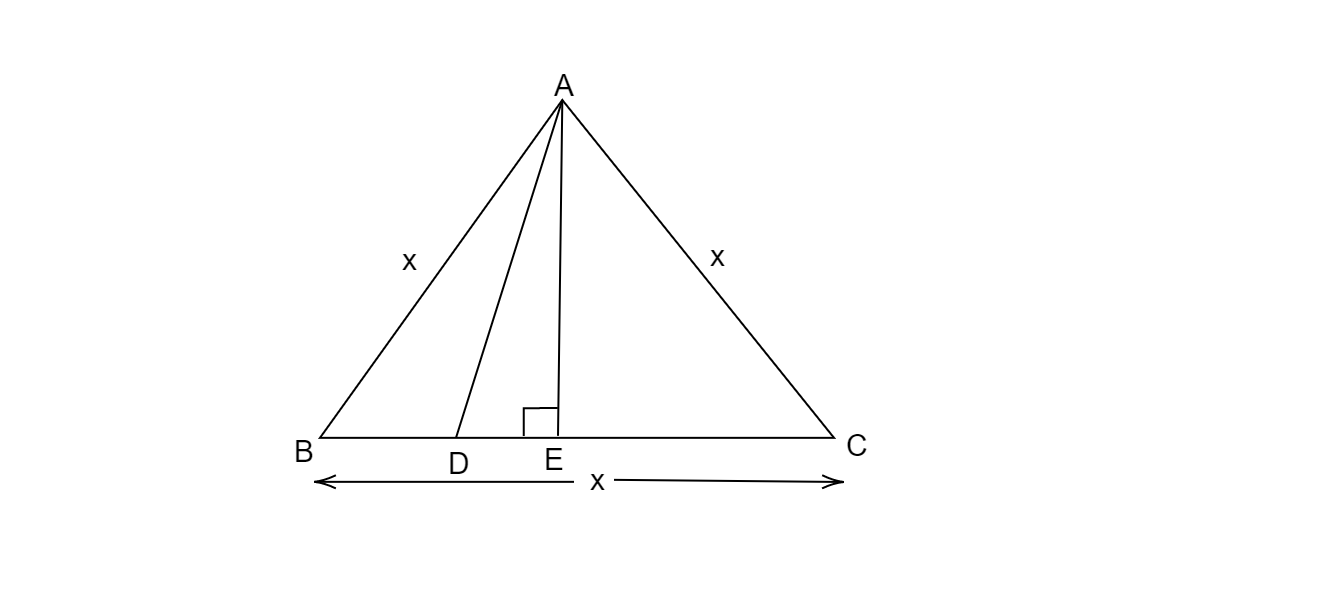# In an equilateral triangle ABC, D is a point on side BC, such that $BD = \frac{1}{3}BC$. Prove that $9AD^2 = 7AB^2$.

Given:

Equilateral $\vartriangle ABC$, D is a such a point on side BC that $BD=\frac{1}{3}BC$.

To do:

We have to prove that $9AD^{2}=7AB^{2}$.

Solution:

Draw $AE\perp BC$.All sides of equilateral; triangles are equal ,

$\therefore AB=BC=CA$

Let $AB=BC=CA=x$

As given

$BD=\frac{1}{3}BC$

$\Rightarrow BD=\frac{x}{3}$

In $\vartriangle AEB$  and $\vartriangle AEC$,

$AE=AE$                      [common]

$AB=AC=x$                      [Equilateral triangle]

$\angle AEB=\angle AEC=90^o$    [$AE\perp BC$]

Therefore, by R.H.S. congruency,

$\vartriangle AEB \cong \vartriangle AEC$

This implies,

$BE=EC$           [C.P.C.T.]

$BE=EC=\frac{x}{2}$

$\Rightarrow BD+DE=\frac{x}{2}$

$\Rightarrow \frac{x}{3}+DE=\frac{x}{2}$

$\Rightarrow DE=\frac{x}{2}-\frac{x}{3}$

$\Rightarrow DE=\frac{x}{6}$

Using Pythagoras Theorem,

$( Hypotenuse)^{2}=( Height)^{2}+( Base)^{2}$

In $\vartriangle AEB$,

$(AB)^{2}=(AE)^{2}+(BE)^{2}$

$x^{2}=(AE)^{2}+( \frac{x}{2})^{2}$

$\Rightarrow (AE)^{2}=x^{2}-\frac{x^{2}}{4}$

$\Rightarrow ( AE)^{2}=\frac{4x^{2}-x^{2}}{4}$

$\Rightarrow ( AE)^2=\frac{3x^{2}}{4}$.........(i)

Similarly, In $\vartriangle AED$

$(AD)^{2}=(AE)^{2}+(DE)^{2}$

$\Rightarrow (AD)^{2}=\frac{3x^{2}}{4}+( \frac{x}{6})^{2}$   [From (i)]

$\Rightarrow (AD)^{2}=\frac{3x^{2}}{4}+\frac{x^{2}}{36}$

$\Rightarrow (AD)^{2}=\frac{27x^{2}+x^{2}}{36}$

$\Rightarrow (AD)^{2}=\frac{28x^{2}}{36}$

$\Rightarrow (AD)^{2}=\frac{7x^{2}}{9}$

$\Rightarrow 9(AD)^{2}=\frac{7x^{2}}{9}\times 9$       [Multiplying both sides by 9]

$\Rightarrow 9(AD)^{2}=7x^{2}$

$\Rightarrow 9(AD)^{2}=7(AB)^{2}$

Hence proved.